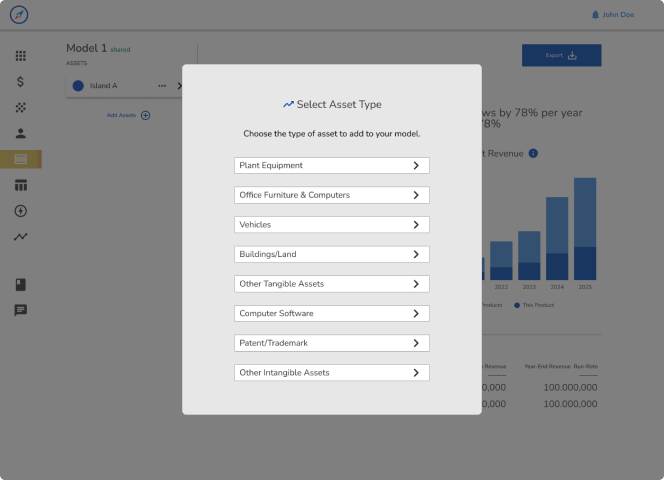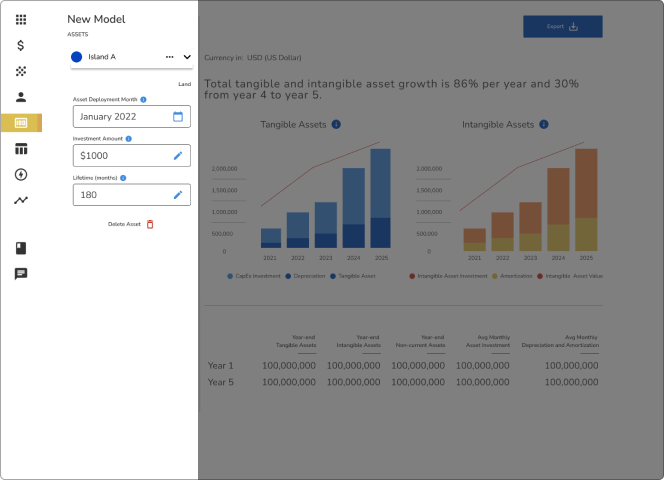# Support and "How To" Guide

### Assets

Assets are investments by the company that hold present or future value. The model automatically calculates short-term assets (cash, accounts receivable, and inventory).

Enter any long-term assets that apply to the model by selecting the type and editing the date, amount, and lifetime over which it will be depreciated. This model assumes a straight-line depreciation method. The model automatically calculates the depreciation/amortization schedule, but it also reinvests at the rate of depreciation. For example, if you purchase a truck and depreciate it over 5 years, the model will depreciate the truck but also reinvest the depreciation amount each month so that the truck is replaced after 5 years.

One-time startup expenses can be capitalized as assets instead of listed as expenses. For example, SaaS companies usually show new software development as an asset investment rather than an expense.

##### Selecting an Asset Type

Add “Assets” to forecast investments in tangible assets (e.g. equipment and land) or intangible assets (e.g. intellectual property and computer software).##### Forecasting Asset Investment

Enter the date (or date range) of the investment, the value, and the depreciation lifetime. The model calculates depreciation and reinvestment to replace the asset.### Asset Inputs

##### Asset Investment Start Month

For Intangible Assets, you can model investment over a period of time. For example, if I am modeling a \$100,000 investment in software development starting in January and deployed in April, the model will show \$25,000 of investment for each of those months. If the asset will be purchased and deployed in a single month, this value should be the same as the Asset Deployment Month.

##### Asset Deployment Month

This is the month the asset will be put into use and begins to depreciate (or amortize). For tangible assets, this is also the month that the asset is purchased and paid for. For Intangible Assets, the investment can take place over a period of time leading up to the Asset Deployment Month.

##### Investment Amount

This is the total amount of money invested in the asset. This amount divided by the lifetime in months will be the amount of depreciation or amortization each month.

This model calculates reinvestment in assets at the same rate as Depreciation or Amortization. For example, if you invest in a truck with a lifetime of 5 years, the model will automatically put away money for the purchase of an identical truck 5 years from now.

If this is an Intangible Asset with an Investment Start Date before the Asset Deployment Date, the Investment Amount will be divided among the months between start and deployment. For example, if I am modeling a \$100,000 investment in software development starting in January and ending in April, the model will show \$25,000 of investment for each of those months.